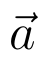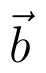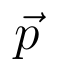Select Page

# CBSE Relations and Functions Maths 12 Science Solutions for MCQ in English

CBSE Relations and Functions Maths 12 Science Solutions for MCQ in English to enable students to get Solutions in a narrative video format for the specific question.

Expert Teacher provides CBSE Relations and Functions Maths 12 Science Solutions for MCQ through Video Solutions in English language. This video solution will be useful for students to understand how to write an answer in exam in order to score more marks. This teacher uses a narrative style for a question from Relations and Functions not only to explain the proper method of answering question, but deriving right answer too.

Please find the question below and view the Solution in a narrative video format.

Question:

Solution Video in English:

You can select video Solutions from other languages also. Please check Solutions in ( Hindi )

## Similar Questions from CBSE, 12th Science, Maths, Relations and Functions

Question 1 : Let R be the relation in the set N given by :
R = {(a, b) : a = b - 2, b > 6}.

Question 2 : Functionsare defined respectively, by, find. (View Answer Video)

Question 3 : A functiondefined asis, (View Answer Video)

Question 4 : If f and g two functions such that they are one-one then g o f is: (View Answer Video)

Question 5 : Number of binary operations on the set { a, b} are: (View Answer Video)

### Linear Programming

Question 1 : The objective function is maximum or minimum, which lies on the boundary of the feasible region. (View Answer Video)

### Vector Algebra

Question 1 : Find the vector quantities from the following:
(i) Time period          (ii) Distance          (iii) Force
(iv) Velocity               (v) Work done

Question 2 :  Find the direction cosines of the vector joining the points A(1, 2, -3) and B(-1, -2, 1) directed from B to A. (View Answer Video)

Question 3 :  Ifanddenote the position vectors of points A and B respectively and C is a point on AB such that AC = 2 CB, then write the position vector of C.  (View Answer Video)

Question 4 : Find the projection of the vectoron the vector(View Answer Video)

Question 5 :  Write the value offor which the vectorsandare parallel vectors.  (View Answer Video)

### Differential Equations

Question 1 : Determine degree of(View Answer Video)

Question 2 :  Find the particular solution of the differential equationgiven thatWhen x =1.  (View Answer Video)

Question 3 : Find the particular solution of the differential equationgiven that y = 0, when x = 0. (View Answer Video)

Question 4 : Solve the differential equationgiven that y = 1 when x = 1. (View Answer Video)

Question 5 : Write the degree of the differential equation :(View Answer Video)